# AP Calculus BC Practice Test 37

### Test Information10 questions20 minutes

Calculator Disallowed

1. If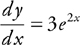and at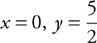, a solution to the differential equation is

2.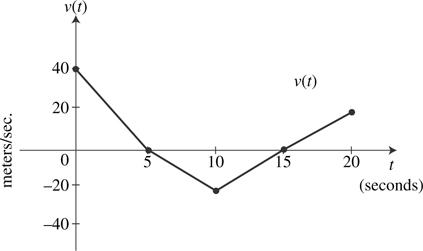The graph of the velocity function of a moving particle is shown above. What is the total displacement of the particle during 0 ≤ t ≤ 20?

3. If h′(x) = k(x) and k is a continuous function for all real values of x, then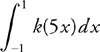is

4. The position function of a moving particle is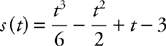for 0 ≤ t ≤ 4. What is the maximum velocity of the particle on the interval 0 ≤ t ≤ 4?

5. Which of the following is an equation of the line tangent to the curve with parametric equations x = 3t2 - 2, y = 2t3 + 2 at the point when t = 1?

6. A function f is continuous on [-1, 1] and some of the values of f are shown below: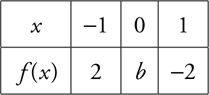If f (x) = 0 has only one solution, r, and r < 0, then a possible value of b is

7.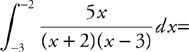8.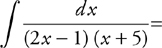9. What is the average value of the function y = 2 sin(2x) on the interval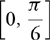?

10. If a particle moves in the xy-plane on a path defined by x = sin2t and y = cos (2t) for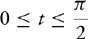, then the length of the arc the particle traces out is